# Discussion on significance ATLAS Statistics Forum CERNPhone 2

• Slides: 15
Download presentation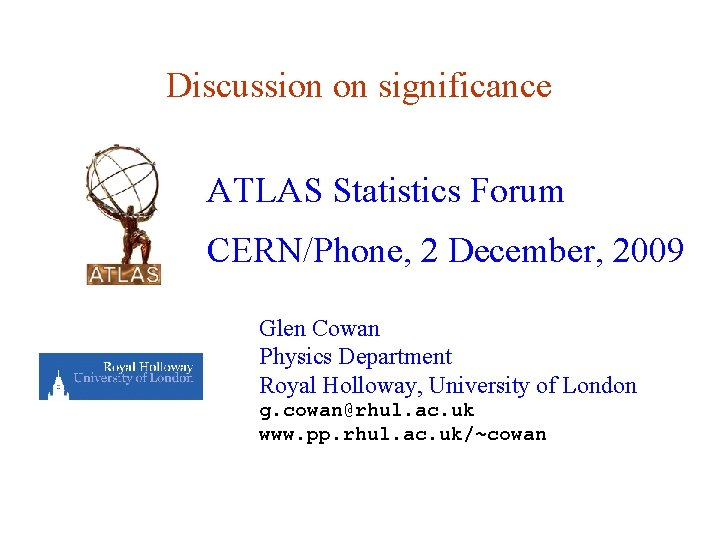Discussion on significance ATLAS Statistics Forum CERN/Phone, 2 December, 2009 Glen Cowan Physics Department Royal Holloway, University of London g. [email protected] ac. uk www. pp. rhul. ac. uk/~cowan G. Cowan, RHUL Physics Discussion on significance page 1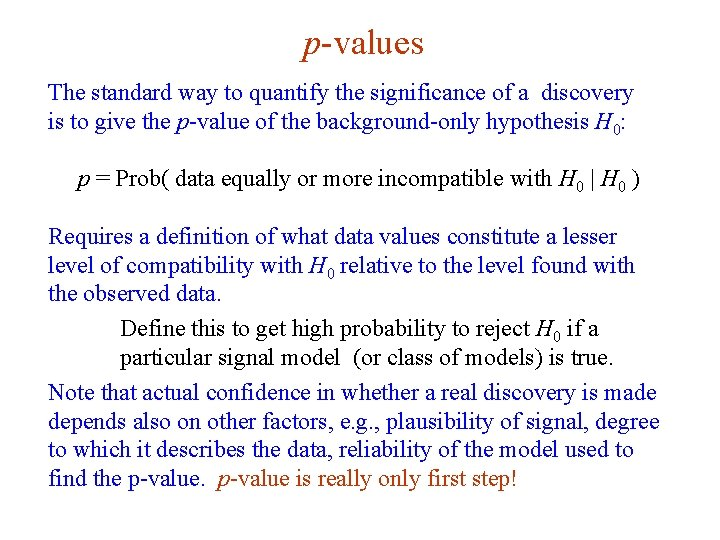p-values The standard way to quantify the significance of a discovery is to give the p-value of the background-only hypothesis H 0: p = Prob( data equally or more incompatible with H 0 | H 0 ) Requires a definition of what data values constitute a lesser level of compatibility with H 0 relative to the level found with the observed data. Define this to get high probability to reject H 0 if a particular signal model (or class of models) is true. Note that actual confidence in whether a real discovery is made depends also on other factors, e. g. , plausibility of signal, degree to which it describes the data, reliability of the model used to find the p-value is really only first step! G. Cowan, RHUL Physics Discussion on significance page 2Significance from p-value Often define significance Z as the number of standard deviations that a Gaussian variable would fluctuate in one direction to give the same p-value. TMath: : Prob TMath: : Norm. Quantile Z = 5 corresponds to p = 2. 87 × 10 -7 G. Cowan, RHUL Physics Discussion on significance page 3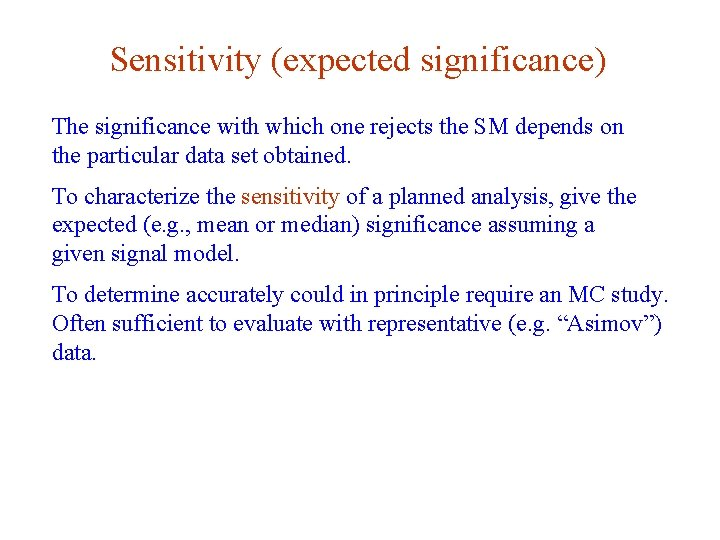Sensitivity (expected significance) The significance with which one rejects the SM depends on the particular data set obtained. To characterize the sensitivity of a planned analysis, give the expected (e. g. , mean or median) significance assuming a given signal model. To determine accurately could in principle require an MC study. Often sufficient to evaluate with representative (e. g. “Asimov”) data. G. Cowan, RHUL Physics Discussion on significance page 4Significance for single counting experiment Suppose we measure n events, expect s signal, b background. n ~ Poisson(s+b) Find p-value of s = 0 hypothesis. data values with n ≥ nobs constitute lesser compatibility G. Cowan, RHUL Physics Discussion on significance page 5Simple counting experiment with LR Equivalently can write expectation value of n as where m is a strength parameter (background-only is m = 0). To test a value of m, construct likelihood ratio where muhat is the Maximum Likelihood Estimator (MLE), which we constrain to be positive: G. Cowan, RHUL Physics Discussion on significance page 6p-value from LR Also define High values correspond to increasing incompatibility with m. For discovery we are testing m = 0. We find The p-value is G. Cowan, RHUL Physics Discussion on significance page 7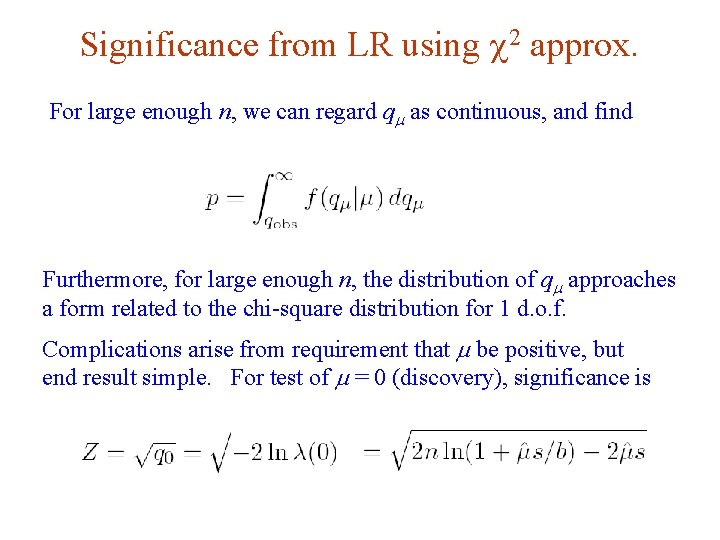Significance from LR using c 2 approx. For large enough n, we can regard qm as continuous, and find Furthermore, for large enough n, the distribution of qm approaches a form related to the chi-square distribution for 1 d. o. f. Complications arise from requirement that m be positive, but end result simple. For test of m = 0 (discovery), significance is G. Cowan, RHUL Physics Discussion on significance page 8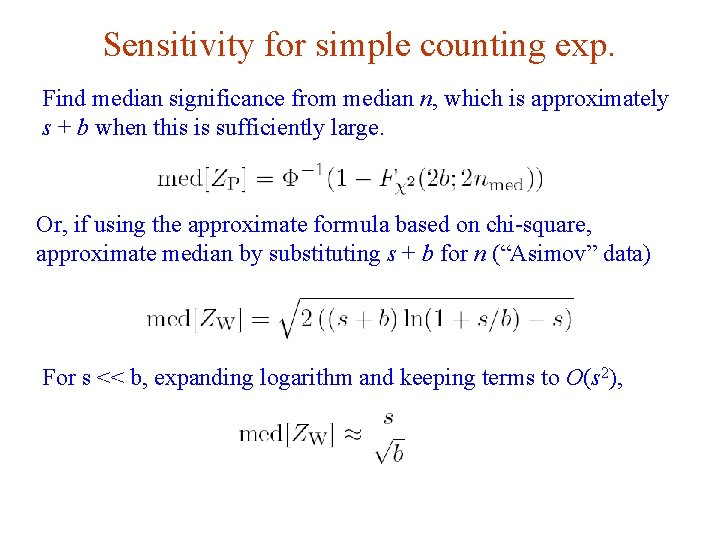Sensitivity for simple counting exp. Find median significance from median n, which is approximately s + b when this is sufficiently large. Or, if using the approximate formula based on chi-square, approximate median by substituting s + b for n (“Asimov” data) For s << b, expanding logarithm and keeping terms to O(s 2), G. Cowan, RHUL Physics Discussion on significance page 9Simple counting exp. with bkg. uncertainty Suppose b consists of several components, and that these are not precisely known but estimated from subsidiary measurements: n ~ Poisson, mi ~ Poisson, Likelihood function for full set of measurements is: G. Cowan, RHUL Physics Discussion on significance page 10Profile likelihood ratio To account for the nuisance parameters (systematics), test m with the profile likelihood ratio: Double hat: maximize L for the given m Single hats: maximize L wrt m and b. Important point is that qm = -2 ln l(m) still related to chi-square distribution even with nuisance parameters (for sufficiently large sample), so retain the simple formula for significance: G. Cowan, RHUL Physics Discussion on significance page 11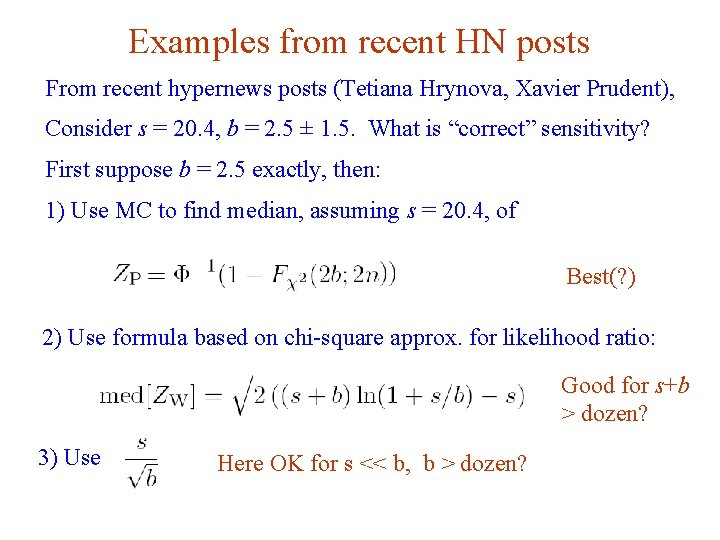Examples from recent HN posts From recent hypernews posts (Tetiana Hrynova, Xavier Prudent), Consider s = 20. 4, b = 2. 5 ± 1. 5. What is “correct” sensitivity? First suppose b = 2. 5 exactly, then: 1) Use MC to find median, assuming s = 20. 4, of Best(? ) 2) Use formula based on chi-square approx. for likelihood ratio: Good for s+b > dozen? 3) Use G. Cowan, RHUL Physics Here OK for s << b, b > dozen? Discussion on significance page 12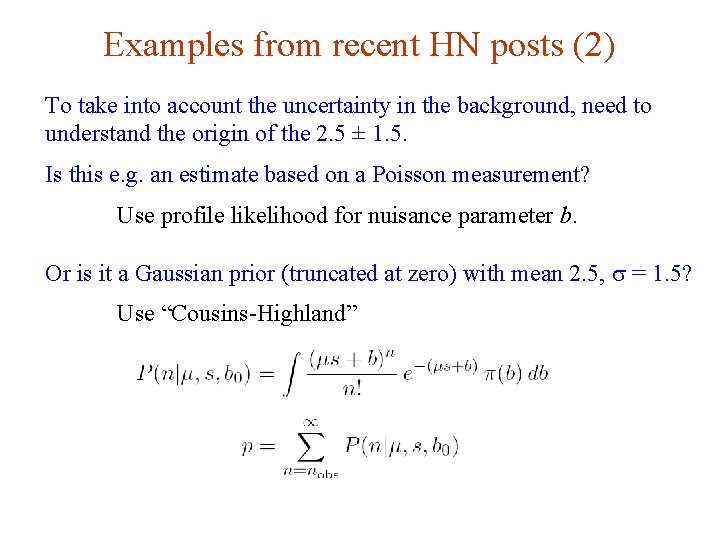Examples from recent HN posts (2) To take into account the uncertainty in the background, need to understand the origin of the 2. 5 ± 1. 5. Is this e. g. an estimate based on a Poisson measurement? Use profile likelihood for nuisance parameter b. Or is it a Gaussian prior (truncated at zero) with mean 2. 5, s = 1. 5? Use “Cousins-Highland” G. Cowan, RHUL Physics Discussion on significance page 13Look-elsewhere effect The p-value should give the probability of rejecting the backgroundonly hypothesis if it is true, i. e. , the probability of a false discovery. But, we carry out many tests, e. g. , we look for a Higgs of many different masses. Need to correct for the fact that the probability that one of these will result in a 5 sigma effect is then > 2. 87 × 10 -7. Several approaches: Treat signal parameter (e. g. Higgs mass) as a floating parameter in the likelihood ratio (Wilks’ thm no good? ) Compute trials factor with MC (find probability that one will reject bkg-only for some (any) point in signal par. space. Approx. correction, e. g. , ~ mass range / mass resolution. Ongoing discussion but should move towards more concrete guidelines. G. Cowan, RHUL Physics Discussion on significance page 14Provisional conclusions Key is to view p-value as the basic quantity of interest; Z is equivalent, and all “magic formulae” are various approximations for Z. Also other considerations for discovery (and limits) beyond p-value, e. g. , level to which signal described by data, plausibility of signal model, reliability of model for p-value, … Also consider e. g. Bayes factors for complementary info. Stat. Forum should move towards firm recommendations on what formulae to use where possible, but cannot investigate every approximation – analysts must take some responsibility here. Draft note (INT) attached to agenda on discovery significance; will also have partner note on limits. G. Cowan, RHUL Physics Discussion on significance page 15